# Newtons laws

## Newton's first law of motion

If no external force acts on a particle, then it is possible to select a set of reference frames, called inertial reference frames, observed from which the particle moves without any change in velocity.

#### In Latin

Lex I: Corpus omne perseverare in statu suo quiescendi vel movendi uniformiter in directum, nisi quatenus a viribus impressis cogitur statum illum mutare. Principia Mathematica.

## Newton's second law of motion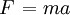$\left. F \right.=ma$

Where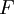$F$ is the force,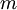$m$ is the mass and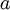$a$ is the acceleration. This law has been found to be true for accelerations as small as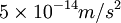$5 \times 10^{-14} m/s^2$ (Ref. 2)

#### In Latin

Lex II: Mutationem motus proportionalem esse vi motrici impressae, et fieri secundum lineam rectam qua vis illa imprimitur. Principia Mathematica.